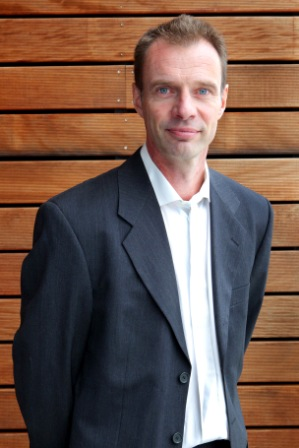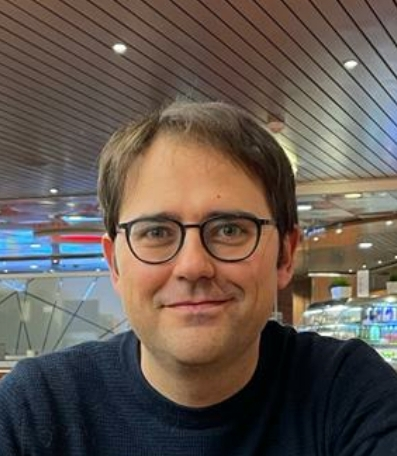# Training Week 13

On 9 - 12 May 2022 NETWORKS organizes the thirteenth Training Week for PhD Students of NETWORKS.

## Minicourse Computational Geometry: Fundamental Techniques

Lecturer: Mark de Berg (TU Eindhoven)

Slides: Networks - mini courseAbstract: In this mini-course I will discuss a number of fundamental results and techniques from computational geometry. One topic will be  Randomized Incremental Construction (RIC), a powerful technique that gives simple and efficient solutions to a variety of algorithmic problems. I will present this technique and discuss several applications of it. Another topic will be so-called arrangements, which arise in motion planning, visibility problems, and many other applications. I will discuss some beautiful tools that are used in the analysis of arrangements, and show several applications.

## Minicourse The Contact Process: recent results on finite-volume phase transitions

Lecturer: Daniel Valesin (University of Warwick)

Slides: Networks - mini courseAbstract: The contact process is a probabilistic model for the spread of an infection in a finite population. In this model, vertices of a graph are individuals, and (unoriented) edges represent proximity between pairs of individuals. At any point in time, each individual may be healthy or infected. Infected individuals recover spontaneously with rate one, but also transmit the infection to each neighbour with a rate equal to lambda, the infectivity parameter.
This model is a type of interacting particle system, a Markov process on a large state space in which the laws of the random evolution are given by simple, local rules. We will start the course with an overview of interacting particle systems, including a summary of classical results for the contact process on infinite lattices and trees.
We will then switch to our main focus of attention: the contact process on finite graphs. In the last two decades, there has been tremendous advance in the study of this process on graphs that capture aspects of real-world populations.  We will cover some highlights of this literature, focusing on the phenomenon of finite-volume phase transition. This means that on many graphs of interest, there exists a critical value lambda_c such that, if the infection rate lambda is below lambda_c, then the infection disappears very quickly from the population, whereas if lambda is above lambda_c, then the infection remains active for a very long time (in many cases, a time that is bounded below by an exponential function of the number of vertices of the graph). This latter, supercritical phase is an instance of metastability, a feature of systems that persist for a long time in a pseudo-equilibrium state (in this case, activity of the infection) until finally moving to the real equilibrium (here, absence of infection).
We will discuss the finite-volume phase transition of the contact process on lattice boxes and truncated regular trees, to illustrate the key methods and ideas. We will then discuss general metastability results that are applicable to large classes of graphs, and go over a few applications, such as the behavior of the contact process on power law random graphs. In the last part of the course we will discuss the contact process on a class of dynamic graphs (the random d-regular graph with switching edges).

Location: Conferentiecentrum De Schildkamp, Leerdamseweg 44, 4147 BM Asperen

Events